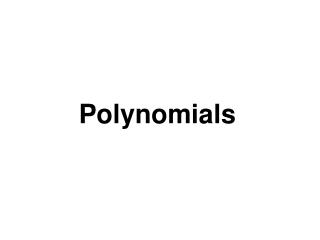# Polynomials - PowerPoint PPT PresentationDownload PresentationPolynomials

PolynomialsDownload Presentation## Polynomials

- - - - - - - - - - - - - - - - - - - - - - - - - - - E N D - - - - - - - - - - - - - - - - - - - - - - - - - - -
##### Presentation Transcript

1. Polynomials

2. The Degree of axn • If a does not equal 0, the degree of axn is n. The degree of a nonzero constant is 0. The constant 0 has no defined degree.

3. Definition of a Polynomial in x • A polynomial in x is an algebraic expression of the form • anxn + an-1xn-1 + an-2xn-2 + … + a1n + a0 • where an, an-1, an-2, …, a1 and a0 are real numbers. an= 0, and n is a non-negative integer. The polynomial is of degree n, an is the leading coefficient, and a0 is the constant term.

4. Text Example Perform the indicated operations and simplify: (-9x3 + 7x2 – 5x + 3) + (13x3 + 2x2 – 8x – 6) Solution (-9x3 + 7x2 – 5x + 3) + (13x3 + 2x2 – 8x – 6) = (-9x3 + 13x3) + (7x2 + 2x2) + (-5x – 8x) + (3 – 6) Group like terms. = 4x3 + 9x2 – (-13x) + (-3) Combine like terms. = 4x3 + 9x2 + 13x – 3

5. Multiplying Polynomials The product of two monomials is obtained by using properties of exponents. For example, (-8x6)(5x3) = -8·5x6+3 = -40x9 Multiply coefficients and add exponents. Furthermore, we can use the distributive property to multiply a monomial and a polynomial that is not a monomial. For example, 3x4(2x3 – 7x + 3) = 3x4 · 2x3 – 3x4 · 7x + 3x4 · 3 = 6x7 – 21x5 + 9x4. monomial trinomial

6. Multiplying Polynomials when Neither is a Monomial • Multiply each term of one polynomial by each term of the other polynomial. Then combine like terms.

7. Using the FOIL Method to Multiply Binomials last first (ax + b)(cx + d) = ax · cx + ax · d + b · cx + b · d Product of First terms Product of Outside terms Product of Inside terms Product of Last terms inner outer

8. Text Example Multiply: (3x + 4)(5x – 3).

9. Text Example Multiply: (3x + 4)(5x – 3). Solution (3x + 4)(5x – 3) = 3x·5x + 3x(-3) + 4(5x) + 4(-3) = 15x2 – 9x + 20x – 12 = 15x2 + 11x – 12 Combine like terms. last first F O I L inner outer

10. The Product of the Sum and Difference of Two Terms • The product of the sum and the difference of the same two terms is the square of the first term minus the square of the second term.

11. The Square of a Binomial Sum • The square of a binomial sum is first term squared plus 2 times the product of the terms plus last term squared.

12. The Square of a Binomial Difference • The square of a binomial difference is first term squared minus 2 times the product of the terms plus last term squared.

13. Special Products Let A and B represent real numbers, variables, or algebraic expressions.  Special ProductExample Sum and Difference of Two Terms (A + B)(A – B) = A2 – B2 (2x + 3)(2x – 3) = (2x) 2 – 32 = 4x2 – 9 Squaring a Binomial (A + B)2 = A2 + 2AB + B2 (y + 5) 2 = y2 + 2·y·5 + 52 = y2 + 10y + 25 (A – B)2 = A2 – 2AB + B2 (3x – 4) 2 = (3x)2 – 2·3x·4 + 42 = 9x2 – 24x + 16 Cubing a Binomial (A + B)3 = A3 + 3A2B + 3AB2 + B3 (x + 4)3 = x3 + 3·x2·4 + 3·x·42 + 43 = x3 + 12x2 + 48x + 64 (A – B)3 = A3 – 3A2B – 3AB2 + B3 (x – 2)3 = x3 – 3·x2·2 – 3·x·22 + 23 = x3 – 6x2 – 12x + 8

14. Text Example Multiply: a. (x + 4y)(3x – 5y) b. (5x + 3y) 2 • Solution • We will perform the multiplication in part (a) using the FOIL method. We will multiply in part (b) using the formula for the square of a binomial, (A + B) 2. • a. (x + 4y)(3x – 5y) Multiply these binomials using the FOIL method. • = (x)(3x) + (x)(-5y) + (4y)(3x) + (4y)(-5y) • = 3x2 – 5xy + 12xy – 20y2 • = 3x2 + 7xy – 20y2Combine like terms. • (5 x + 3y) 2 = (5 x) 2 + 2(5 x)(3y) + (3y) 2 (A + B) 2 = A2 + 2AB + B2 • = 25x2 + 30xy + 9y2 F O I L

15. Example • Multiply: (3x + 4)2. Solution: ( 3x + 4 )2=(3x)2 + (2)(3x) (4) + 42=9x2 + 24x + 16

16. Polynomials